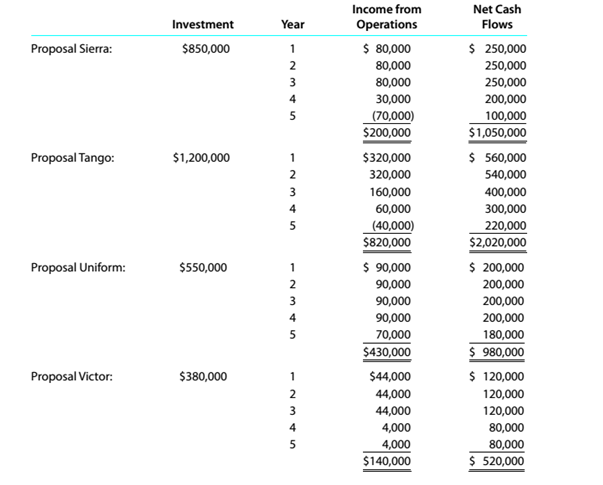Chapter 15, Problem 15.6.8P

Chapter
Section
Textbook Problem

Capital rationing decision involving four proposalsKopecky Industries Inc. is considering allocating a limited amount of capital investmentfunds among four proposals. The amount of proposed investment, estimated income fromoperations, and net cash flow for each proposal are as follows:The company’s capital rationing policy requires a maximum cash payback period of three years. In addition, a minimum average rate of return of 12% is required on all projects. 1f the preceding standards are met, the net present value method and present value indexes are used to rank the remaining proposals. InstructionsBased on the analyses, comment on the relative attractiveness of the proposals ranked inparts (6) and (7).

To determine

Concept Introduction:

NPV:

Net present value (NPV) is the method to evaluate the project feasibility. This method calculates the present value of cash inflows and outflows, and then calculates the net present value of the investment. A project should be accepted if it has a positive NPV. The formula to calculate the NPV is as follows:

NPV = Present value of cash inflows  Present value of cash out flows

To Indicate:

The Comment on the Ranking for the proposals

Explanation

The Ranking for the proposals is determined as follows:

 Net Present Value Present Value Index Rank

Still sussing out bartleby?

Check out a sample textbook solution.

See a sample solution

The Solution to Your Study Problems

Bartleby provides explanations to thousands of textbook problems written by our experts, many with advanced degrees!

Get Started

Find more solutions based on key concepts